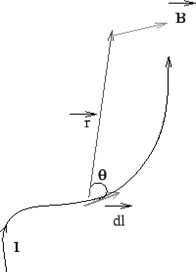Biot- Savarts' Law: Biot-Savarts' law provides an expression for the magnetic field due to a current segment. The fieldat a positiondue to a current segmentis experimentally found to be perpendicular toand. The magnitude of the field is

• proportional to the lengthand to the currentand to the sine of the angle betweenand.
• inversely proportional to the square of the distanceof the point P from the current element.In SI units the constant of proportionality is, whereis the permeability of the free space. The value ofis• The expression for field at a point P having a position vectorwith respect to the current element isFor a conducting wire of arbitrary shape, the field is obtained by vectorially adding the contributions due to such current elements as per superposition principle,where the integration is along the path of the current flow.